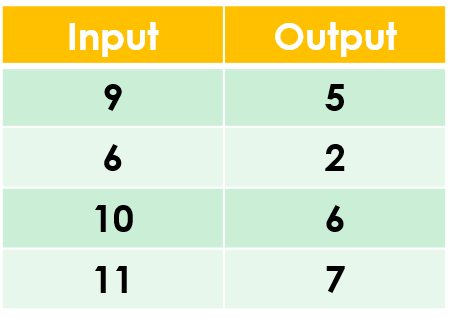1
visibility

Find the rule from the table below:• A

Subtract 3

• B

Subtract 4

The correct answer is "Subtract 4".

Subtraction is the operation that decreases a number by taking away from it.

Solution:
To find the correct rule, we consider the given table.

We observe that the given rule makes each number decrease. This implies subtraction.

We try subtracting 4 from each input number:

9 - 4 = 5
6 - 4 = 2
10 - 4 = 6
11 - 4 = 7

On subtracting 4 from the input numbers, we get the corresponding output numbers.

So, the rule is “subtract 4”.